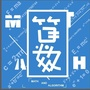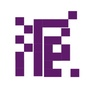0+0+0+0+0+0+0+1+0+0+0+0+0+0+0+0+0+0+0+7+0+0+3+0+0+0+0+0+2+0+0+2+0+0+0+0+0+0+0+0+2+0+0+4+0+
Top